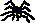Errata

#### Functionality, Polymorphism, and Concurrency:A Mathematical Investigation of Programming Paradigms

• Lemma 2.11: Add two equations "1s=s" and "1k=k".

• Proof of Theorem 2.18: For completeness, let T be the theory generated by E, and observe...

• Remark 2.20: ...then Th(A) is a lambda theory. Moreover, the properties of Lemma 2.11 imply that s and k, and hence all combinatory terms, are lambda-definable, which implies that A is a lambda algebra.

• Section 2.6.2: ...e.g. the open term algebra of the lambda-beta-eta-calculus is extenional...

• Proposition 4.4: Let (P,*) be an ordered applicative structure, where P is a bounded tree and * is strict in its left argument.

• Corollary 4.5: Begin with a tree P and a monotone left-strict binary operation...

• Section 5.2.1: In the definition of raw typed lambda terms, omit "M sigma".

Back to Homepage:Peter Selinger / Department of Mathematics and Statistics / Dalhousie University
selinger@mathstat.dal.ca / PGP key